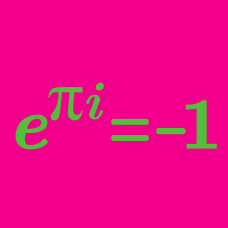Algebra

# De Moivre's Theorem: Level 3 Challenges

$\large { z }^{ 3 }=1$

What is the set of all $$z$$ that satisfy the equation above?

Note: $$\omega = \frac{ -1 + \sqrt 3 i}{2}$$ where $$i =\sqrt{-1}$$.

Find the value of $(2-\omega)(2-\omega^2)(2-\omega^{10})(2-\omega^{11}).$

Details and Assumptions:

$$\omega$$ is a non-real cube root of unity.

The five roots of the equation $$z^{5}=4-4i$$ each take the form

$\Large \sqrt{2} e ^ { \frac{ k \pi i } { 20} },$

where $$k$$ is a positive integer less than 40.

Find the sum of all values of $$k$$.

$\large x + \frac 1 x = \sqrt 3\ , \ \ \ \ \ \ \ x^{200} + \frac {1}{x^{200}} = \ ?$

If the $$6$$ solutions of $$x^{6}=-64$$ are written in the form $$a+ib$$, where $$a$$ and $$b$$ are real, then what is the product of those solutions with $$a>0$$?

×

Problem Loading...

Note Loading...

Set Loading...